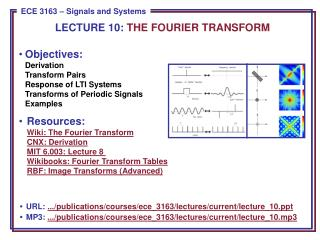# Objectives: Derivation Transform Pairs Response of LTI Systems Transforms of Periodic Signals Examples - PowerPoint PPT PresentationDownload PresentationObjectives: Derivation Transform Pairs Response of LTI Systems Transforms of Periodic Signals Examples

Objectives: Derivation Transform Pairs Response of LTI Systems Transforms of Periodic Signals Examples
Download Presentation## Objectives: Derivation Transform Pairs Response of LTI Systems Transforms of Periodic Signals Examples

- - - - - - - - - - - - - - - - - - - - - - - - - - - E N D - - - - - - - - - - - - - - - - - - - - - - - - - - -
##### Presentation Transcript

1. LECTURE 10: THE FOURIER TRANSFORM • Objectives:DerivationTransform PairsResponse of LTI SystemsTransforms of Periodic SignalsExamples • Resources:Wiki: The Fourier TransformCNX: DerivationMIT 6.003: Lecture 8 Wikibooks: Fourier Transform TablesRBF: Image Transforms (Advanced) • URL: .../publications/courses/ece_3163/lectures/current/lecture_10.ppt • MP3: .../publications/courses/ece_3163/lectures/current/lecture_10.mp3

2. Motivation • We have introduced a Fourier Series for analyzing periodic signals. What about aperiodic signals? (e.g., a pulse instead of a pulse train) • We can view an aperiodic signal as the limit of a periodic signal as T . • The harmonic components are spaced apart. • As T ,0 0, then k0 becomes continuous. • The Fourier Series becomes the Fourier Transform.

3. Derivation of Analysis Equation • Assume x(t) has a finite duration. • Define as a periodic extensionof x(t): • As • Recall our Fourier series pair: • Since x(t) andare identical over this interval: • As

4. Derivation of the Synthesis Equation • Recall: • We can substitute for ckthe sampled value of : • As • and we arrive at our Fourier Transform pair: • Note the presence of the eigenfunction: • Also note the symmetry of these equations (e.g., integrals over time and frequency, change in the sign of the exponential, difference in scale factors). (synthesis) (analysis)

5. Frequency Response of a CT LTI System • Recall that the impulse response ofa CT system, h(t), defines the properties of that system. • We apply the Fourier Transform toobtain the system’s frequency response: • except that now this is valid for finite duration (energy) signals as well as periodic signals! • How does this relate to what you have learned in circuit theory? CT LTI CT LTI

6. Existence of the Fourier Transform • Under what conditions does this transform exist? • x(t) can be infinite duration but must satisfy these conditions:

7. Example: Impulse Function

8. Example: Exponential Function

9. Example: Square Pulse

10. Example: Gaussian Pulse

11. CT Fourier Transforms of Periodic Signals

12. Example: Cosine Function

13. Example: Periodic Pulse Train

14. Summary • Motivated the derivation of the CT Fourier Transform by starting with the Fourier Series and increasing the period: T  • Derived the analysis and synthesis equations (Fourier Transform pairs). • Applied the Fourier Transform to CT LTI systems and showed that we can obtain the frequency response of an LTI system by taking the Fourier Transform of its impulse response. • Discussed the conditions under which the Fourier Transform exists. Demonstrated that it can be applied to periodic signals and infinite duration signals as well as finite duration signals. • Worked several examples of important finite duration signals. • Introduced the Fourier Transform of a periodic signal. • Applied this to a cosinewave and a pulse train.